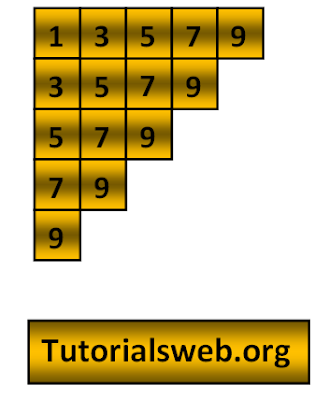## Write "C"  program to print below Number pattern 16:To understand this example, you should have knowledge of the following topics:
·      C programming operators
C Number pattern 16 program using For loop:
```
#include <stdio.h>
int main()
{
int i,j;
for(i=1;i<=9;i+=2)
{
for(j=i;j<=9;j+=2)
{
printf("%d",j);
}
printf("\n");
}
return 0;
}
```
Output:
`13579`
`3579`
`579`
`79`
`9`
Explanation:
• First two integers "i", and "j" are declared of type int.
• Then in the First for loop “i” value is initialized with a value 9.
• Now “i” value is checked with the condition i <= 9 that is (1 <= 9). So the condition is True.
• Now the loop enters into second for loop and checks the condition j <= 9. Where "j = 1". and the condition is True print the value of "j".
• The value of "j" prints up to condition becomes False.
• Now the value of "i" will be incremented by the condition "i = i+2" and executes the loop.
• when the value of "i"  becomes "11" the program terminates.